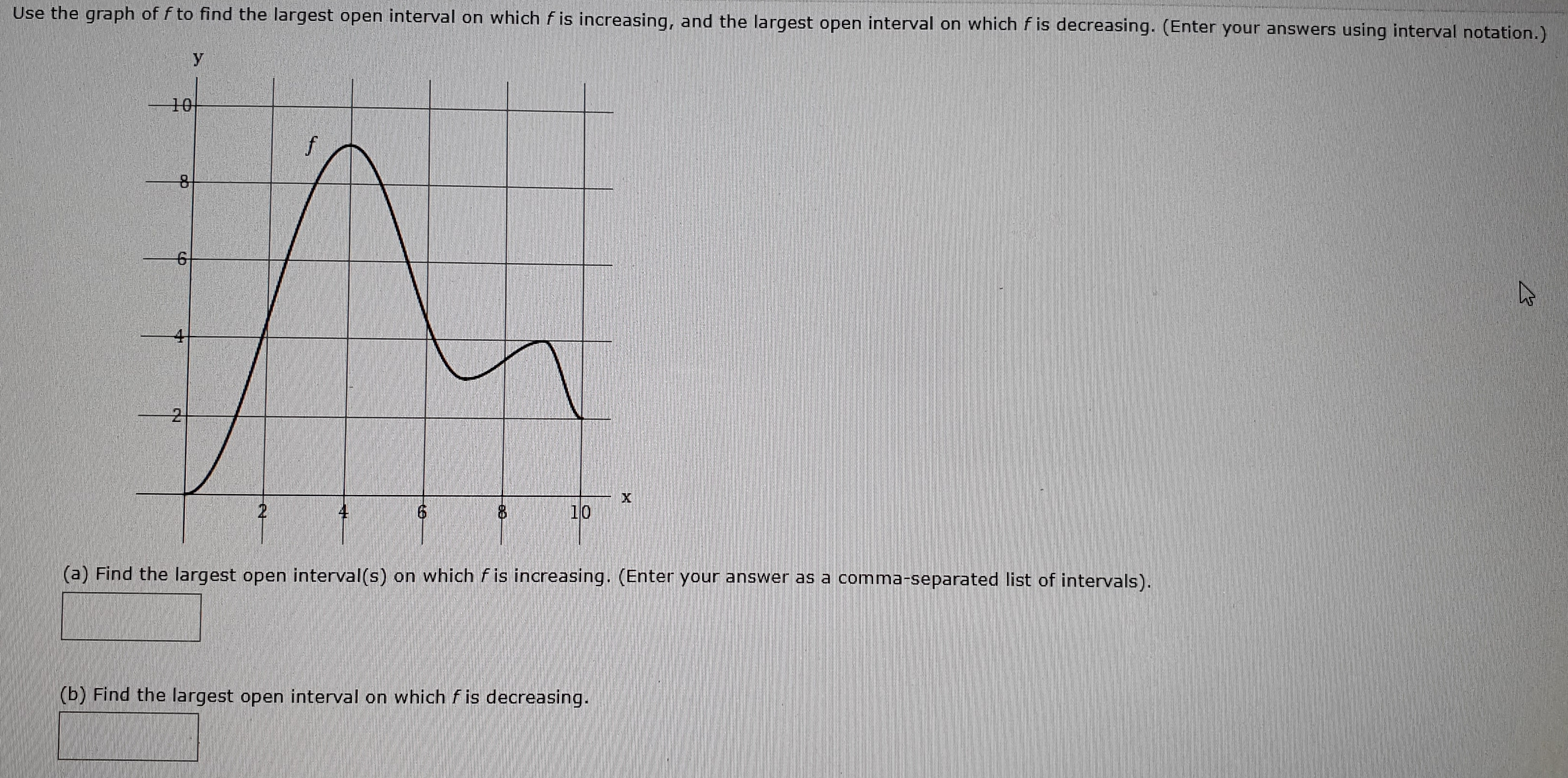# Use the graph of f to find the largest open interval on which f is increasing, and the largest open interval on which f is decreasing. (Enter your answers using interval notation.)10f102(a) Find the largest open interval(s) on which fis increasing. (Enter your answer as a comma-separated list of intervals).(b) Find the largest open interval on which fis decreasing.X

Question
565 views

How can I get the result?

Which is the result?help_outlineImage TranscriptioncloseUse the graph of f to find the largest open interval on which f is increasing, and the largest open interval on which f is decreasing. (Enter your answers using interval notation.) 10 f 10 2 (a) Find the largest open interval(s) on which fis increasing. (Enter your answer as a comma-separated list of intervals). (b) Find the largest open interval on which fis decreasing. X fullscreen
check_circle

Step 1

Please see the picture on the white board. I have used the picture in the question and marked all the popints of inflections of the curve y = f(x). Please note points A, B, C, D & E.

Step 2

By observation, the function y = f(x) is:

• Increasing from point A to B
• Decreasing from B to C
• Increasing from C to D
• Decreasing from D to E
Step 3

Part (a)

Again by observation, interval A to B is larger than C to D.

Hence, the largest interval in which f is increasing is A to B. An...

### Want to see the full answer?

See Solution

#### Want to see this answer and more?

Solutions are written by subject experts who are available 24/7. Questions are typically answered within 1 hour.*

See Solution
*Response times may vary by subject and question.
Tagged in

### Functions